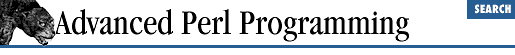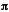#Chapter 4Subroutine References and Closures## 4.2 Using Subroutine References

Let's look at some common examples of using subroutine references: callback functions and higher-order procedures.

A callback function is an ordinary subroutine whose reference is passed around. The caller (who uses that reference) doesn't necessarily have an idea of which subroutine is getting invoked. Let's examine three simple examples involving callback functions: dispatch tables, signal handlers, and plotting functions.

### 4.2.1 Dispatch Table

A typical dispatch table is an array of subroutine references. The following example shows %options as a dispatch table that maps a set of command-line options to different subroutines:

```%options = (       # For each option, call appropriate subroutine.
"-h"         => \&help,
"-f"         => sub {\$askNoQuestions = 1},
"-r"         => sub {\$recursive = 1},
"_default_"  => \&default,
);

ProcessArgs (\@ARGV, \%options); # Pass both as references```

Some of these references in this code are to named subroutines. Others don't do much, so it is just simpler to code them as inline, anonymous subroutines. ProcessArgs can now be written in a very generic way. It takes two arguments: a reference to an array that it parses and a mapping of options that it refers to while processing the array. For each option, it calls the appropriate "mapped" function, and if an invalid flag is supplied in @ARGV, it calls the function corresponding to the string _default_.

ProcessArgs is shown in Example 4.1.

#### Example 4.1: ProcessArgs

```ProcessArgs (\@ARGV, \%options); # Pass both as references
sub ProcessArgs {
# Notice the notation: rl = ref. to array, rh = ref. to hash
my (\$rlArgs, \$rhOptions) = @_;
foreach \$arg (@\$rlArgs) {
if (exists \$rhOptions->{\$arg}) {
# The value must be a reference to a subroutine
\$rsub = \$rhOptions->{\$arg};
&\$rsub();   # Call it.
} else {        #option does not exist.
if (exists \$rhOptions->{"_default_"}) {
&{\$rhOptions{"_default_"}};
}
}
}
}```

You can omit one step by using the block form of dereferencing (hark back to Section 1.2.5, "A More General Rule"), like this:

```if (exists \$rhOptions->{\$arg}) {
&{\$rhOptions->{\$arg}}(); # Dereference and call sub in one shot
}```

I prefer the more verbose version for its readability.

### 4.2.2 Signal Handlers

Usually, a program works by calling functions implemented by the operating system, not vice versa. An exception to this rule is when the operating system has an urgent message to deliver to the program. In many operating systems, the delivery is accomplished by means of signals. A signal might be issued, for example, when a user presses Ctrl-C, when a floating-point exception is trapped by the hardware, or when a child process dies. You can specify a function to be called whenever a signal is delivered to your program. This allows you to take appropriate action. A Ctrl-C handler, for example, might perform clean-up before exiting. A floating-point exception handler might set an error flag and resume normal operation.

Perl provides a convenient way to specify signal handlers for each type of signal. There's a special variable called %SIG whose keys are the names of signals, and its values correspond to subroutine names or references, which are called for the corresponding signal.

```sub ctrl_c_handler  {
print "Ctrl C pressed \n";
}
\$SIG {"INT"} = \&ctrl_c_handler;  # "INT" indicates  "Interrupt"
# signal. ```

Here, the word INT is a reserved string and signifies keyboard interrupts with Ctrl-C. Your operating system's documentation for signals will tell you the names of signals that might be sent to your program or script. In fact, you can get this information from Perl also by asking it to print out some of its configuration information:

```use Config; # Load the Config module
print \$Config{sig_name};```

When you assign values to %SIG, Perl also allows you to give the name of the subroutine, so you don't have to give it a subroutine reference:

`\$SIG {"INT"} = 'ctrl_c_handler';  # Name of the subroutine passed.`

Incidentally, signal handlers are fraught with peril. Perl internally uses C library functions such as malloc, which are not reentrant. If a signal handler is triggered just when such a function is being called and the signal handler also happens to call the same function, the function gets totally confused and is likely to crash the system. This behavior is even more insidious at the script level, because you have no idea when Perl might call malloc. (Chapter 20, Perl Internals, should give you a very good idea.) The moral of the story is that you should attempt to do the least possible work in a signal handler, such as set a previously defined global variable to true, and check this variable's value in the code outside.

#### 4.2.2.1 Expression plotting

Suppose we want to plot a variety of functions, of the general type:

`y = f(x)`

where `f(x)` is a function that takes a number as an argument and returns another number. Examples include sin(x), cos(x), and sqrt(x). But in addition to such simple examples, we would like to be able to plot arbitrarily complex expressions such as

`y = sin(2x) + cos2(x); `

It is easy to develop a subroutine plot that can plot this expression in the range 0 to 2:

```\$PI = 3.1415927;
\$rs = sub {                           # Anonymous subroutine
my(\$x) = @_;
return sin (2*\$x) + cos(\$x) ** 2; # Function to be plotted
};
plot (\$rs, 0, 2 * \$PI, 0.01);```

This is an example of a higher-order procedure that takes (a reference to) another user-defined subroutine as an input parameter and calls it one or more times. sort is an example of a built-in higher-order procedures; the difference is that it takes subroutine names, not references.